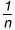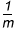# Activities

••• ##### Subject Area

• Math: Elementary Math: Fractions

• ##### AuthorK-5

30 Minutes

• ##### Device
•TI-Nspire™ CX/CX II
•TI-Nspire™ CX CAS/CX II CAS
• ##### Software

TI-Nspire™
TI-Nspire™ CAS

## Fraction Multiplication

#### Activity Overview

This activity uses interactive unit squares to explore the concept of fraction multiplication. The concept of fraction multiplication is developed by considering the area of a unit square that has been partitioned into rectangles.

This TI-Nspire™ lesson uses interactive unit squares to explore the concept of fraction multiplication. The concept of fraction multiplication is developed by considering the area of a unit square that has been partitioned into rectangles with dimensionsby.  Building on earlier work of finding the area of a rectangle by multiplying side lengths, students investigate the fraction of an area shaded in a unit square. This leads to the generalization that the product of two fractions is the product of the numerators and the product of the denominators.